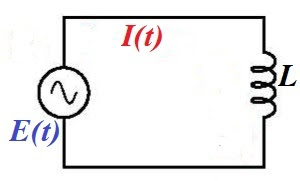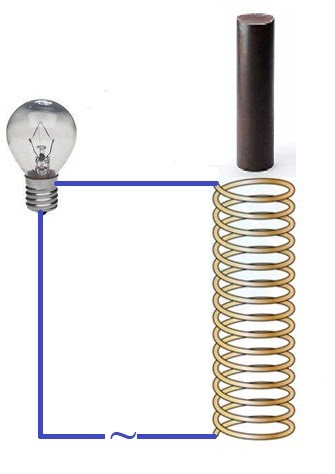## Friday, October 2, 2020

### AC Inductors: UNIZOR.COM - Physics4Teens - Electromagnetism

Notes to a video lecture on http://www.unizor.com

Alternating Current and Inductors

For the purpose of this lecture it's important to be familiar with the concept of a self-induction explained in "Electromagnetism - Self-Induction" chapter of this course.

In this lecture we will discuss the AC circuit that contains an inductor - a wire wound in a reel or a solenoid, thus making multiple loops, schematically presented on the following picture.Both direct and alternating current go through an inductor, but, while
direct current goes with very little resistance through a wire, whether
it's in a shape of a loop or not, alternating current meets some
additional resistance when this wire is wound into a loop.

Consider the following experiment.Here the AC circuit includes a lamp, an inductor in a shape of a solenoid and an iron rod fit to be inserted into a solenoid.

While the rod is not inside a solenoid, the lamp lights with normal
intensity. But let's gradually insert an iron core into a solenoid. As
the core goes deeper into a solenoid, the lamp produces less and less
light, as if some kind of resistance is increasing in the circuit.

This experiment demonstrates that inductors in the AC circuit produce
effect similar to resistors, and the more "inductive" the inductor - the
more resistance can be observed in a circuit.

The theory behind this is explained in this lecture.

The cause of this resistance is self-induction. This concept was
explained earlier in this course and its essence is that variable
magnetic field flux, going through a wire loop, creates electromotive
force (EMF) directed against the original EMF that drives electric
current through a loop.

Any current that goes along a wire creates a magnetic field around this
wire. Since the current in our wire loop is alternating, the magnetic
field that goes through this loop is variable. According to the
Faraday's Law, the variable magnetic field going through a wire loop
generates EMF equal to a rate of change of the magnetic field flux and
directed opposite to the EMF that drives the current through a wire,
thus resisting it.

Magnetic flux Φ(t) going through inductor, as a function of time t, is proportional to an electric current I(t) going through its wire

Φ(t) = L·I(t)

where L is a coefficient of proportionality that depends
on physical properties of the inductor (number of loop in a reel, type
of its core etc.) called inductance of the inductor.

If the current is alternating as

I(t) = Imax·sin(ωt)

the flux will be

Φ(t) = L·Imax·sin(ωt)

According to Faraday's Law, self-induction EMF Ei
is equal in magnitude to a rate of change of magnetic flux and opposite
in sign (see chapter "Electromagnetism - "Self-Induction" in this
course)

Ei(t) = −dΦ/dt =

= −L·
dI(t)/dt =

= −L·ω·Imax·cos(ωt) =

= −L·ω·Imax·sin(ωt+π/2) =

= −Eimax·sin(ωt+π/2)

where

Eimax = L·ω·Imax

The unit of measurement of inductance is henry (H) with 1H being an inductance of an inductor that generates 1V electromotive force, if the rate of change of current is 1A/sec.

That is,

henry = volt·sec/ampere = ohm·sec

An expression XL=L·ω in the above formula for Ei is called inductive reactance. It plays the same role for an inductor as resistance for resistors.

The units of the inductive reactance is Ohm (Ω) because

henry/sec = ohm·sec/sec = ohm.

Using this concept of inductive reactance XL of an inductor, the time dependent induced EMF is

Ei(t) = −XL·Imax·sin(ωt+π/2) = −Eimax·sin(ωt+π/2)

and

Eimax = XL·Imax,

which for inductors in AC circuit is an analogue of the Ohm's Law for resistors.

What's most important in the formula

Ei(t) = −Eimax·sin(ωt+π/2)

and the most important property of an inductor in an AC circuit is
that, while the electric current in a circuit oscillates with angular
speed ω, the voltage drop on an inductor oscillates with the same angular speed ω as the current, but its period is shifted in time by π/2 relative to the current.